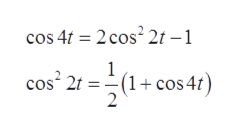# |17. f(1) = cos² 21 17. cos? 21 = (1+ cos 47); [s-1 +s/(s² + 16)].

Question
2 views

Have both question and answer (second picture), but need help knowing how to get that answer using laplace transform methods

check_circle

Step 1

The given function,

Step 2

Using the formula in the following,help_outlineImage Transcriptionclosecos 4t = 2 cos² 2t – 1 (1+ cos 41) cos 2t = fullscreen
Step 3

Therefore...

### Want to see the full answer?

See Solution

#### Want to see this answer and more?

Solutions are written by subject experts who are available 24/7. Questions are typically answered within 1 hour.*

See Solution
*Response times may vary by subject and question.
Tagged in

### Math